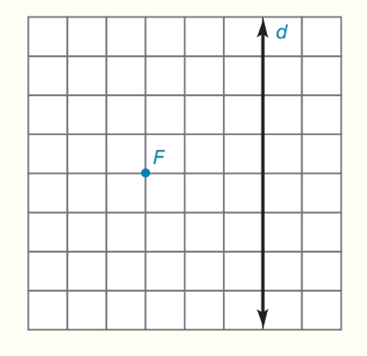Chapter 7.CT, Problem 17CTElementary Geometry For College St...

7th Edition
Alexander + 2 others
ISBN: 9781337614085

Solutions

Chapter
SectionElementary Geometry For College St...

7th Edition
Alexander + 2 others
ISBN: 9781337614085
Textbook Problem

Sketch the parabola that has directrix d and focus F.To determine

To sketch:

The parabola that has directrix d and focus F.

Explanation

Calculation:

A parabola is the locus of points that are equidistant t from a fixed line and a fixed point not on that line.

Given:

Draw FAd at point A. Note that FA is the axis of symmetry of the parabola. Locate the midpoint of FA, which is also the vertex V of the parabola.

Through F, draw a line parallel to d. With a compass, measure the distance FA and mark off arcs of that length on the parallel line (on both sides of F)

Still sussing out bartleby?

Check out a sample textbook solution.

See a sample solution

The Solution to Your Study Problems

Bartleby provides explanations to thousands of textbook problems written by our experts, many with advanced degrees!

Get Started

Solve the equations in Exercises 126. 2(x21)x2+1x4x2+1x2+1=0

Finite Mathematics and Applied Calculus (MindTap Course List)

In Exercises 13 and 14, find the inverse function of f. f(x)=83x3

Calculus: An Applied Approach (MindTap Course List)

Find the limit. limt2t24t38

Single Variable Calculus: Early Transcendentals

Solve for x: ln(e + x) = 1. a) 0 b) 1 c) e d) ee e

Study Guide for Stewart's Single Variable Calculus: Early Transcendentals, 8th